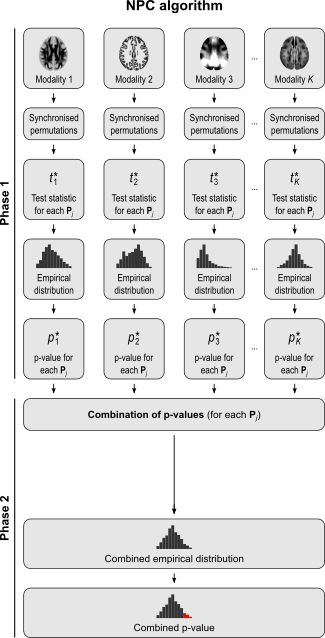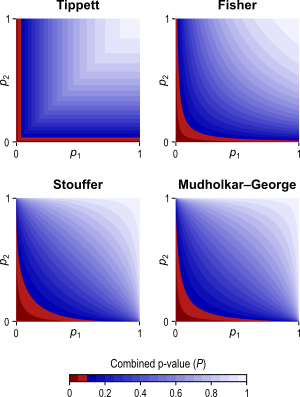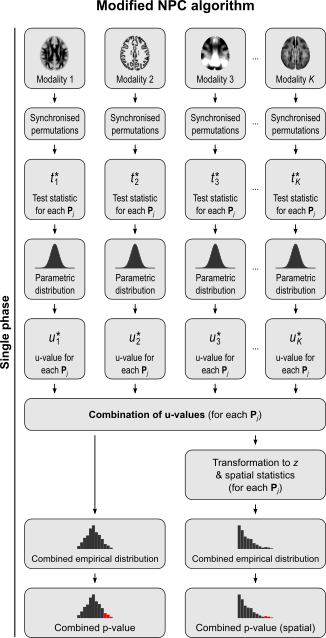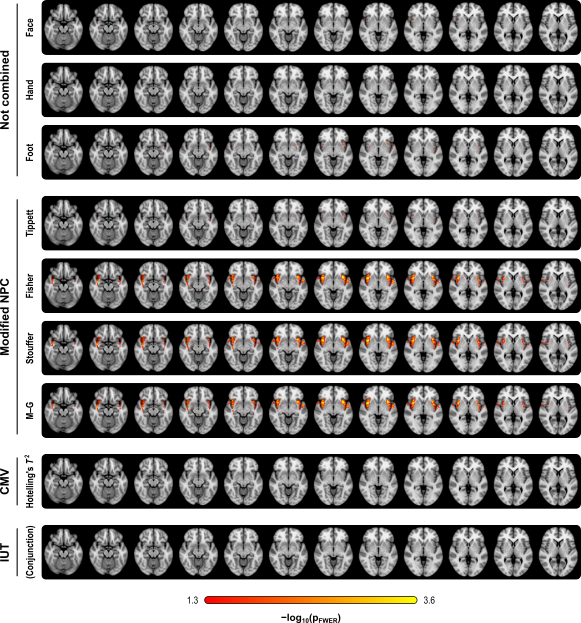# Non-Parametric Combination (NPC) for brain imaging

Have you ever had an analysis in which there was a large set of contrasts, all of interest, and you were worried about multiple testing? An eventual effect would be missed by a simple Bonferroni correction, but you did not know what else to do? Or did you have a set of different studies and you wished to obtain a style of meta-analytic result, indicating whether there would be evidence across all of them, without requiring the studies to be all consistently significant?

The Non-Parametric Combination (NPC) solves these issues. It is a way of performing joint inference on multiple data collected on the same experimental units (e.g., same subjects), all with minimal assumptions. The method was proposed originally by Pesarin (1990, 1992) [see references below], independently by Blair and Karninski (1993), and described extensively by Pesarin and Salmaso (2010). In this blog entry, the NPC is presented in brief, with emphasis on the modifications we introduce to render it feasible for brain imaging. The complete details are in our paper that has just been published in the journal Human Brain Mapping.

## NPC in a nutshell

The NPC consists of, in a first phase, testing each hypothesis separately using permutations that are performed synchronously across datasets; these tests are termed partial tests. The resulting statistics for each and every permutation are recorded, allowing an estimate of the complete empirical null distribution to be constructed for each one. In a second phase, the empirical p-values for each statistic are combined, for each permutation, into a joint statistic. As such a combined joint statistic is produced from the previous permutations, an estimate of its empirical distribution function is immediately known, and so is the p-value of the joint test. A flowchart of the original algorithm is shown below; click to see it side-by-side with the modified one (described below).## A host of combining functions

The null hypothesis of the NPC is that null hypotheses for all partial tests are true, and the alternative hypothesis that any is false, which is the same null of a union-intersection test (UIT; Roy, 1953). The rejection region depends on how the combined statistic is produced. Various combining functions, which produce such combined statistics, can be considered, and some of the most well known are listed in the table below:

Method Statistic p-value
Tippett$\min \left(p_{k}\right)$$1-\left(1-T\right)^{K}$
Fisher$-2 \sum_{k=1}^{K} \ln\left(p_{k}\right)$$1-\chi^{2}\left(T;\;\nu=2K\right)$
Stouffer$\frac{1}{\sqrt{K}} \sum_{k=1}^{K} \Phi^{-1}\left(1-p_{k}\right)$$1-\Phi\left(T;\;\mu=0,\;\sigma^2=1\right)$
Mudholkar–George$\frac{1}{\pi}\sqrt{\frac{3(5K+4)}{K(5K+2)}}\sum_{k=1}^{K} \ln\left(\frac{1-p_{k}}{p_{k}}\right)$$1-t_{\text{cdf}}(T;\;\nu=5K+4)$

In the table,$K$ is the number of partial tests, and the remaining of the variables follow the usual notation (see the Table 1 in the paper for the complete description). Many of these combining functions were proposed over the years for applications such as meta-analyses, and many of them assume independence between the tests being combined, and will give incorrect p-values if such assumption is not met. In the NPC, lack of dependence is not a problem, even if these same functions are used: the synchronised permutations ensure that any dependence, if existing, is taken into account, and this is done so implicitly, with no need for explicit modelling.

The different combining functions lead to different rejection regions for the null hypothesis. For the four combining functions in the table above, the respective rejection regions are in the figure below.The combining functions can be modified to allow combination of tests so as to favour hypotheses with concordant directions, or be modified for bi-directional tests. Click on the figure above for examples of these cases (again, see the paper for the complete details).

## Two problems, one solution

The multiple testing problem is well known in brain imaging: as an image comprises thousands of voxels/vertices/faces, correction is necessary. Bonferroni is in general too conservative, and various other approaches have been proposed, such as the random field theory. Permutation tests provide control over the familywise error rate (FWER) for the multiple tests across space, requiring only the assumption of exchangeability. This is all well known; see Nichols and Hayasaka (2003) and Winkler et al. (2014) for details.

However, another type of multiple testing is also common: analyses that test multiple hypotheses using the same model, multiple pairwise group comparisons, multiple and distinct models, studies using multiple modalities, that mix imaging and non-imaging data, that consider multiple processing pipelines, and even multiple multivariate analyses. All these common cases also need multiple testing correction. We call this multiple testing problem MTP-II, to discern it from the well known multiple testing problem across space, described above, which we term MTP-I.

One of the many combining functions possible with NPC, the one proposed by Tippett (1931), has a further property that makes it remarkably interesting. The Tippett function uses the smallest p-value across partial tests as its test statistic. Alternatively, if all statistics are comparable, it can be formulated in terms of the maximum statistic. It turns out that the distribution of the maximum statistic across a set of tests is also the distribution that can be used in a closed testing procedure (Marcus et al., 1976) to correct for the familywise error rate (FWER) using resampling methods, such as permutation. In the context of joint inference, FWER-correction can also be seen as an UIT. Thus, NPC offers a link between combination of multiple tests, and correction for multiple tests, in both cases regardless of any dependence between such tests.

This means that the MTP-II, for which correction in the parametric realm is either non-existing or fiendishly difficult, can be accommodated easily. It requires no explicit modelling of the dependence between the tests, and the resulting error rates are controlled exactly at the test level, adding rigour to what otherwise could lead to an excess of false positives without correction, or be overly conservative if a naïve correction such as Bonferroni were attempted.

## Modifying for imaging applications

As originally proposed, in practice NPC cannot be used in brain imaging. As the statistics for all partial tests for all permutations need to be recorded, an enormous amount of space for data storage is necessary. Even if storage space were not a problem, the discreteness of the p-values for the partial tests is problematic when correcting for multiple testing, because with thousands of tests in an image, ties are likely to occur, further causing ties among the combined statistics. If too many tests across an image share the same most extreme statistic, correction for the MTP-I, while still valid, becomes less powerful (Westfall and Young, 1993; Pantazis et al., 2005). The most obvious workaround — run an ever larger number of permutations to break the ties — may not be possible for small sample sizes, or when possible, requires correspondingly larger data storage.

The solution is loosely based on the direct combination of the test statistics, by converting the test statistics of the partial tests to values that behave as p-values, using the asymptotic distribution of the statistics for the partial tests. We call these as “u-values”, in order to emphasise that they are not meant to be read or interpreted as p-values, but rather as transitional values that allow combinations that otherwise would not be possible.

For spatial statistics, the asymptotic distribution of the combined statistic is used, this time to produce a z-score, which can be subjected to the computation of cluster extent, cluster mass, and/or threshold-free cluster enhancement (TFCE; Smith and Nichols, 2009). A flow chart of the modified algorithm is shown below. Click to see it side-by-side with the original.## More power, fewer assumptions

One of the most remarkable features of NPC is that the synchronised permutations implicitly account for the dependence structure among the partial tests. This means that even combining methods originally derived under the assumption of independence can be used when such independence is untenable. As the p-values are assessed via permutations, distributional restrictions are likewise not necessary, liberating NPC from most assumptions that thwart parametric methods in general. This renders NPC a good alternative to classical multivariate tests, such as MANOVA, MANCOVA, and Hotelling’s T2 tests: each of the response variables can be seen as an univariate partial test in the context of the combination, but without the assumptions that are embodied in these old multivariate tests.

As if all the above were not already sufficient, NPC is also more powerful than such classical multivariate tests. This refers to its finite sample consistency property, that is, even with fixed sample size, as the number of modalities being combined increases, the power of the test also increases. The power of classical multivariate tests, however, increases up to a certain point, then begins to decrease, eventually reaching zero when the number of combining variables match the sample size.

The figure below summarises the analysis of a subset of the subjects of a published FMRI study (Brooks et al, 2005) in which painful stimulation was applied to the face, hand, and foot of 12 subjects. Using permutation tests separately, no results could be identified for any of the three types of stimulation. A simple multivariate test, the Hotelling’s T2 test, even assessed using permutations, did not reveal any effect of stimulation either. The NPC results, however, suggest involvement of large portions of the anterior insula and secondary somatosensory cortex. The Fisher, Stouffer and Mudholkar–George combining functions were particularly successful in recovering a small area of activity in the midbrain and periaqueductal gray area, which would be expected from previous studies on pain, but that could not be located from the original, non-combined data.Detailed assessment of power, using variable number of modalities, and of modalities containing signal, is shown in the paper.

## Combinations or conjunctions?

Combination, as done via NPC, is different than conjunctions (Nichols et al., 2005) in the following: in the combination, one seeks for aggregate significance across partial tests, without the need that any individual study is necessarily significant. In the conjunction, it is necessary that all of them, with no exception, is significant. As indicated above, the NPC forms an union-intersection test (UIT; Roy, 1953), whereas the conjunctions form an intersection-union test (IUT; Berger, 1982). The former can be said to be significant if any (or an aggregate) of the partial tests is significant, whereas the latter is significant if all the partial tests are.

## Availability

The NPC, with the modifications for brain imaging, is available in the tool PALM — Permutation Analysis of Linear Models. It runs in either Matlab or Octave, and is free (GPL).

## References

Contributed to this post: Tom Nichols.